# Comparison of Test Data and CFD

The mean velocity field is determined by time averaging over five complete runner revolutions for the hybrid models and eight complete runner revolutions for the URANS models to filter out all unsteadiness. The survey axes, ${S*}$, at sections W0 – W2 (see Fig. 1), are normalized by the throat radius, ${R_{throat}}$= 0.05m, and the velocity is normalized by the bulk velocity at the throat, ${W_{throat}}$. The axial axis is downward and the runner rotates in the positive direction according to the right-hand rule.

Figure 8 compares the axial and tangential velocity distributions of the high-Reynolds number turbulence models, standard ${k-\epsilon }$, SST ${k-\omega }$, realizable ${k-\epsilon }$and RNG ${k-\epsilon }$, with the experimental results at W1 and W2. It is shown that the turbulence models predict the mean velocity very similarly. It is known that a high level of swirl leads to an on-axis recirculation region due to the high centrifugal force . The models overestimate the size of that region, although the RNG ${k-\epsilon }$and standard ${k-\epsilon }$models predict the recirculation region more realistically than the other models. Javadi et al.  used the RNG ${k-\epsilon }$model in the swirl generator over a wide range of runner rotational speeds, from part load to full load. They concluded that the applicability of the RNG ${k-\epsilon }$model is restricted to a range close to the best efficiency point. As mentioned before, the current flow field resembles the Francis turbine at 70% load, which is far from the best efficiency point. The disintegration process of the vortex rope is highly unsteady and needs to be resolved to capture its realistic representation. The turbulence models reproduce the width of the central stagnant region reasonably well at W2.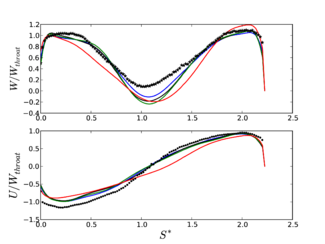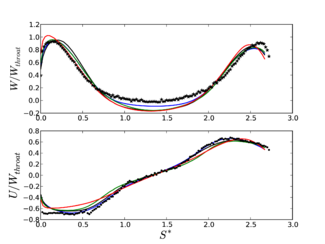Figure 8: Axial and tangential velocity with high-Reynolds number turbulence models at W1. Black: RNG ${k-\epsilon }$. Blue: standard ${k-\epsilon }$. Green: realizable ${k-\epsilon }$. Red: SST ${k-\omega }$. Star markers (*): experimental axial velocity. Point markers (${\bullet }$): experimental tangential velocity. Left: W1 and right: W2.

Figure 9 compares the axial and tangential velocity distributions of the low-Reynolds number turbulence models, Launder-Sharma and Lien-cubic ${k-\epsilon }$, with the experimental results at W1. The results show that Lien-cubic ${k-\epsilon }$underestimates the main character of the flow, the on-axis recirculation region, considerably. It can be seen that Launder-Sharma ${k-\epsilon }$model, on the other hand, overestimates the recirculation region. The LS model was initially proposed for predicting swirling flows. The model, as a modified version of the standard ${k-\epsilon }$model, is one of the earliest and most widely used models for resolving the near-wall flow behavior. Although some damping functions are added in the LS model to account for the viscous and wall effects, the improved near-wall behavior does not significantly increase the quality of the mean velocity profiles in the draft tube.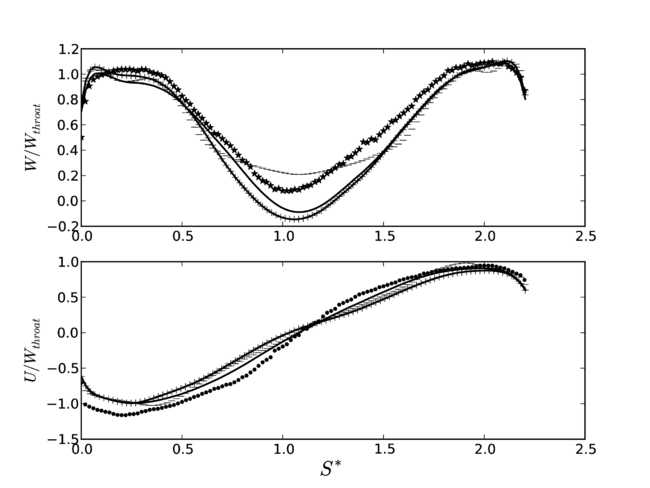Figure 9: Axial and tangential velocity with low-Reynolds number turbulence models compared with experimental results at W1. Solid line: LS with an inlet boundary condition extracted from high-Reynolds number simulation. Solid line with + markers: LS with constant inlet boundary condition. Dashed line: LC. Star markers (*): experimental axial velocity. Point markers (${\bullet }$): experimental tangential velocity.

Figure 10 compares the axial and tangential velocity distributions of the hybrid models DDES-SA, IDDES-SA and DDES-SST, with the experimental results at sections W0 – W2. Figure 10a shows that the velocity increases linearly from the hub to the shroud downstream of the runner, at W0. The blade wakes are responsible for the change in the slope of velocity components at ${0.25. The wakes travel closer to the hub, i.e. the velocity components decrease in the inner half of the annular cross-section. The hybrid models realistically predict the inner half of the flow, close to the hub. The axial velocity in the outer part, close to the shroud, is overestimated and the tangential velocity is underestimated, i.e. the predicted flow is too axial in the outer part, at W0. The effects of the wakes on the averaged velocity is overestimated by DDES-SA and IDDES-SA. Nevertheless, the wakes predicted by these two models are remarkably in spite of while a different wall treatment being used by IDDES-SA. DDES-SST presents weaker wakes compared with the other models while the results agree with the experimental results. Figure 8b shows the axial and tangential velocity components at W1, close to the throat. As mentioned before, the vortex breakdown leads to a disintegration of the helical vortex. The vortex rope forms slightly downstream the nozzle and immediately deflects outwards, see Figs 12 and 14. The on-axis axial velocity is almost zero and the tangential velocity increases linearly with the radius. In the outer part of the draft tube, both the axial and tangential velocity magnitudes increase at W1. The central recirculation region thus ends at the throat, yielding an on-axis stagnation region at W1. The experimental results show ${W/W_{throat}}$= 0.08 on-axis at W1 while numerical results predict ${W/W_{throat}}$= -0.010, -0.041 and -0.187 with DDES-SA, DDES-SST and IDDES-SA, respectively. Figure 10c shows the axial and tangential velocity components at W2. The central cone-shaped stagnant region in the draft tube occupies 60% of the cross-section and is thus much wider than at W1. The velocity profiles at W2 follow the same patterns as at W1 but with a lower magnitude.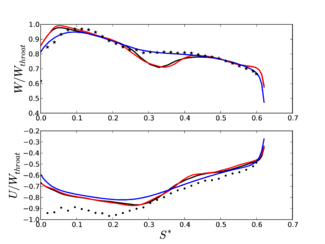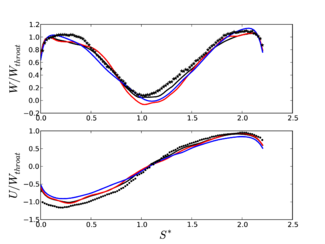(a) W0 (b) W1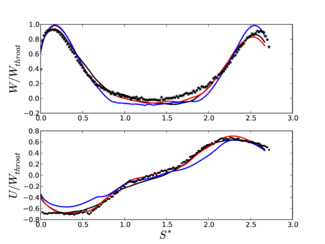(c) W2 Figure 10: Axial and tangential velocity distributions of the hybrid URANS-LES models, compared with the experimental results. Black: DDES-SA. Red: IDDES-SA. Blue: DDES-SST. Star markers (*): experimental axial velocity. Point markers (${\bullet }$): experimental tangential velocity.

Figure 11 shows the resolved root mean square of the axial and tangential velocity fluctuations at W0 and W1, ${w_{rms}^{'}}$and ${u_{rms}^{'}}$, respectively. The results of DDES-SA and the RNG ${k-\epsilon }$models are compared with the experimental results. The modeled part of the turbulence is different model to model, ${(u_{rms}^{'},w_{rms}^{'})_{modeled}/W_{throat}=0.1}$at both W0 and W1 for DDES-SA, and ${(u_{rms}^{'},w_{rms}^{'})_{modeled}/W_{throat}=0.02}$at W0 and ${(u_{rms}^{'},w_{rms}^{'})_{modeled}/W_{throat}=0.14}$at W1 for the RNG ${k-\epsilon }$model. Therefore, the total turbulence predicted by DDES-SA is slightly higher than the experimental results for both components at W0 and is closer to the experimental results at W1. The ratio of modeled to total part of the turbulence at the peaks are almost 50% at W0 and 15% at W1 for DDES-SA. The RNG ${k-\epsilon }$model still underestimates the turbulence at W0 and W1. At W0, ${w_{rms}^{'}}$has a single experimental peak at ${S^{*}}$= 0.25, which is predicted at ${S^{*}}$= 0.3 by DDES-SA.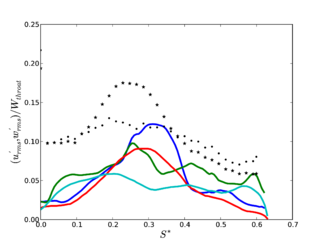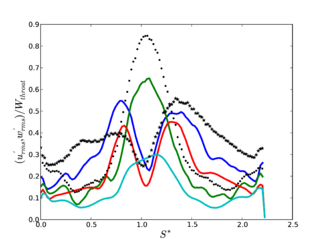(a) W0 (b) W1 Figure 11: Axial , ${w_{rms}^{'}}$, and tangential, ${u_{rms}^{'}}$, root mean square velocity fluctuations with DDES-SA compared with the experimental results. Green: DDES-SA, ${w_{rms}^{'}}$. Blue: DDES-SA, ${u_{rms}^{'}}$. Red: RNG ${k-\epsilon }$, ${w_{rms}^{'}}$. Turquoise: RNG ${k-\epsilon }$, ${u_{rms}^{'}}$. Star markers (*): experimental ${w_{rms}^{'}}$. Point markers (${\bullet }$): experimental ${u_{rms}^{'}}$.

Figure 12 shows that the blade wakes are responsible for this peak. The numerical result is quantitatively close to the experimental results. The experimental tangential velocity fluctuation, ${u_{rms}^{'}}$, increases moderately from hub to shroud, while the numerical profile suggests a more pronounced effect of the wakes. At W1, the axial velocity fluctuation, ${w_{rms}^{'}}$, has two peaks, while the tangential velocity fluctuation, ${u_{rms}^{'}}$, has a single peak. The two peaks of the axial velocity fluctuation are predicted reasonably well, which confirms that DDES-SA is able to resolve the turbulent structures very well. These two peaks are related to the shear layer between the inner stagnant region and the outer flow, which is the source of most of the turbulence in the draft tube. The total ${w_{rms}^{'}}$predicted by DDES-SA is slightly higher than the experimental results, if the right peak of the profile at ${S^{*}\sim 1.4}$is considered as the reference. The total ${w_{rms}^{'}}$predicted by the RNG ${k-\epsilon }$model agrees excellently with the experimental results. The peak of the tangential velocity fluctuation can be related to the swirl in the on-axis recirculation region. DDES-SA is capable of predicting the high level of turbulence production of ${u_{rms}^{'}}$, yielding an increase of ${u_{rms}^{'}}$by a factor of seven between W0 and W1. The RNG ${k-\epsilon }$model still underpredicts the on-axis peak of ${u_{rms}^{'}}$.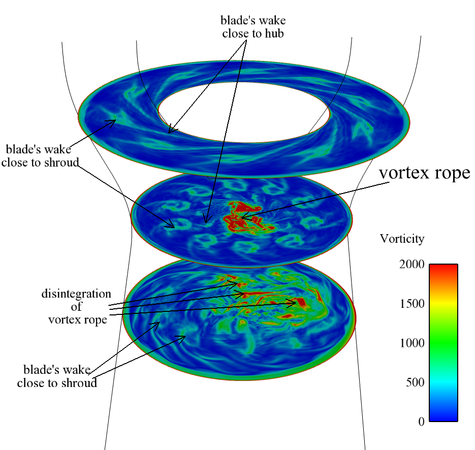Figure 12: Vorticity magnitude with DDES-SA at three horizontal planes in the draft tube

Figure 12 shows the vorticity magnitude predicted by DDES-SA at three planes in the draft tube. It is worth mentioning that the wakes are tenuous compared with the vortex rope in the draft tube. If DDES-SA is considered as the reference results, the RNG ${k-\epsilon }$gives a smaller inter-blade region with lower vorticity and a larger wake with higher vorticity compared with LS. Thus, the RNG ${k-\epsilon }$model can be interpreted as an eddy-viscosity model which predicts better blade wakes.

Figure 13 shows the iso-surface of the pressure, representing the vortex rope captured by the standard ${k-\epsilon }$, RNG ${k-\epsilon }$, LS and DDES-SA models. It can be seen that standard ${k-\epsilon }$and RNG ${k-\epsilon }$models predict very similar vortex ropes. The LS model predicts a vortex rope which is axially more compact than other eddy-viscosity models. DDES-SA predicts the most detailed vortex rope. Thus, DDES-SA predicts a higher axial surge of the vortex rope.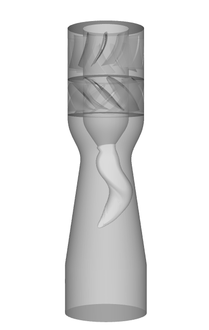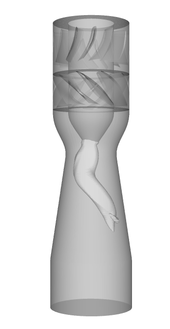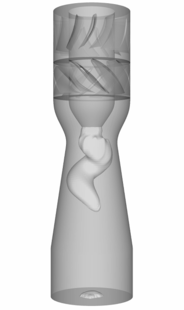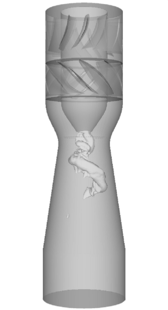a) b) c) d) Figure 13: Iso-surface of pressure, visualizing the vortex rope, a) standard ${k-\epsilon }$, b) RNG ${k-\epsilon }$, c) LS, d) DDES‑SA.

Figure 14 shows an iso-surface of the ${q}$-criterion by DDES-SA. The separations and the consecutive coherent structures form after the leading edge at the suction side close to the hub and move radially outward due to the pressure gradient which is caused by the swirl. As can be seen in Fig. 12, the structures are stronger at the outer part of the blade. The blade wakes are deflected toward the hub due to the divergent part of the draft tube. Figure 14 also shows the disintegration of the vortex rope. Further downstream, the wakes and the vortex rope come together, and the precessing vortex rope ruptures the structures of the wakes. It is noteworthy that the inner part of the wake attaches and then detaches to/from the nozzle in the draft tube. This forms a small recirculation region nested downstream of the nozzle in the draft tube.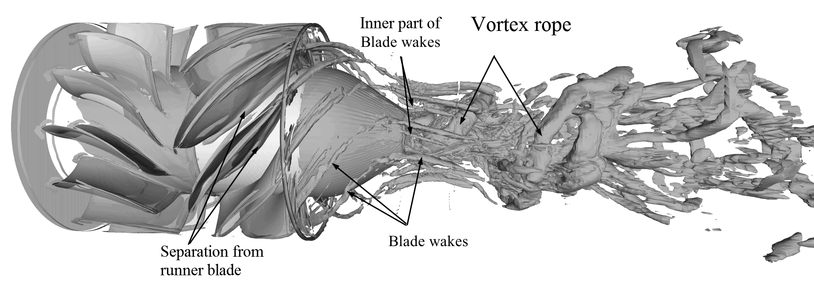Figure 14: Iso-surface of ${q}$-criterion, DDES-SA

Contributed by: A. Javadia, A. Bosiocb, H Nilssona, S. Munteanc, R. Susan-Resigab — aChalmers University of Technology, Göteborg, Sweden; bUniversity Polytehnica Timişoara, Timişoara, Romania; cCenter for Advanced Research in Engineering Sciences, Romanian Academy, Timişoara Branch, Timişoara, Romania